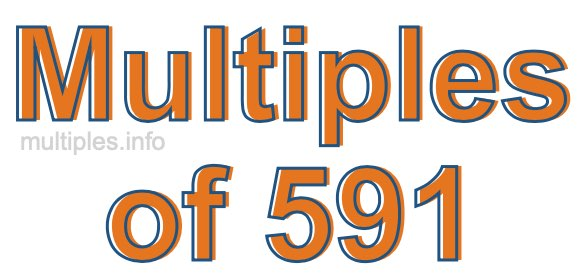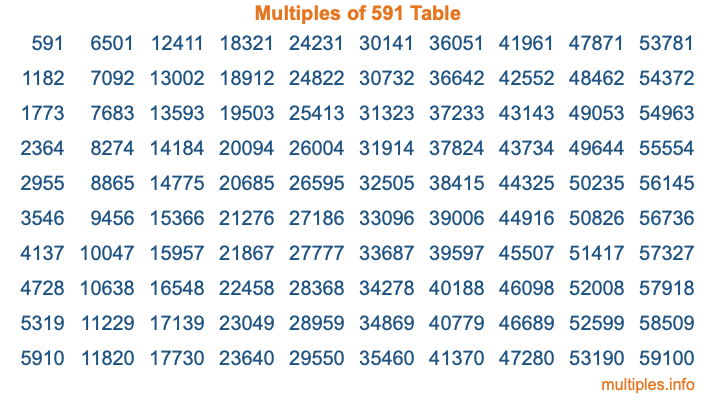Multiples of 591Welcome to the Multiples of 591 page. Here we will first teach you everything you will ever need to know about the multiples of 591, and then give you a study guide summary of everything we taught you to make sure you remember it all. Use this page to look up facts and learn information about the multiples of 591. This page will make you a multiples of five hundred ninety-one expert!

Definition of Multiples of 591
Multiples of 591 are all the numbers that when divided by 591 equal an integer. Each of the multiples of 591 are called a multiple. A multiple of 591 is created by multiplying 591 by an integer.

Therefore, to create a list of multiples of 591, you start with 1 multiplied by 591, then 2 multiplied by 591, then 3 multiplied by 591, and so on for as long as you want. Thus, the list of the first five multiples of 591 is 591, 1182, 1773, 2364, and 2955. To see a larger list of multiples of 591, see the printable image of Multiples of 591 further down on this page. We also have a category where you can choose any nth multiple of 591.

Multiples of 591 Checker
The Multiples of 591 Checker below checks to see if any number of your choice is a multiple of 591. In other words, it checks to see if there is any number (integer) that when multiplied by 591 will equal your number. To do that, we divide your number by 591. If the the quotient is an integer, then your number is a multiple of 591.

Is  a multiple of 591?

Least Common Multiple of 591 and ...
A Least Common Multiple (LCM) is the lowest multiple that two or more numbers have in common. This is also called the smallest common multiple or lowest common multiple and is useful to know when you are adding our subtracting fractions. Enter one or more numbers below (591 is already entered) to find the LCM.

Check out our LCM Calculator if you need more details about the Least Common Multiple or if you need the LCM for different numbers for adding and subtraction fractions.

nth Multiple of 591
As we stated above, 591 is the first multiple of 591, 1182 is the second multiple of 591, 1773 is the third multiple of 591, and so on. Enter a number below to find the nth multiple of 591.

th multiple of 591

Multiples of 591 vs Factors of 591
591 is a multiple of 591 and a factor of 591, but that is where the similarities end. All postive multiples of 591 are 591 or greater than 591. All positive factors of 591 are 591 or less than 591.

Below is the beginning list of multiples of 591 and the factors of 591 so you can compare:

Multiples of 591: 591, 1182, 1773, 2364, 2955, etc.

Factors of 591: 1, 3, 197, 591

As you can see, the multiples of 591 are all the numbers that you can divide by 591 to get a whole number. The factors of 591, on the other hand, are all the whole numbers that you can multiply by another whole number to get 591.

It's also interesting to note that if a number (x) is a factor of 591, then 591 will also be a multiple of that number (x).

Multiples of 591 vs Divisors of 591
The divisors of 591 are all the integers that 591 can be divided by evenly. Below is a list of the divisors of 591.

Divisors of 591: 1, 3, 197, 591

The interesting thing to note here is that if you take any multiple of 591 and divide it by a divisor of 591, you will see that the quotient is an integer.

Multiples of 591 Table
Below is an image of the first 100 multiples of 591 in a table. The table is in chronological order, column by column. The first column has the first ten multiples of 591, the second column has the next ten multiples of 591, and so on.The Multiples of 591 Table is also referred to as the 591 Times Table or Times Table of 591. You are welcome to print out our table for your studies.

Negative Multiples of 591
Although not often discussed or needed in math, it is worth mentioning that you can make a list of negative multiples of 591 by multiplying 591 by -1, then by -2, then by -3, and so on, to get the following list of negative multiples of 591:

-591, -1182, -1773, -2364, -2955, etc.

Multiples of 591 Summary
Below is a summary of important Multiples of 591 facts that we have discussed on this page. To retain the knowledge on this page, we recommend that you read through the summary and explain to yourself or a study partner why they hold true.

There are an infinite number of multiples of 591.

A multiple of 591 divided by 591 will equal a whole number.

591 divided by a factor of 591 equals a divisor of 591.

The nth multiple of 591 is n times 591.

The largest factor of 591 is equal to the first positive multiple of 591.

591 is a multiple of every factor of 591.

591 is a multiple of 591.

A multiple of 591 divided by a divisor of 591 equals an integer.

591 divided by a divisor of 591 equals a factor of 591.

Any integer times 591 will equal a multiple of 591.

Multiples of a Number
Here you can get the multiples of another number, all with the same attention to detail as we did for multiples of 591 on this page.

Multiples of
Multiples of 592
Did you find our page about multiples of five hundred ninety-one educational? Do you want more knowledge? Check out the multiples of the next number on our list!